# Ride comfort performance of heavy truck with three control cases of semi-active isolation systems

## Van Liem Nguyen1, Van Quynh Le2

1, 2Hubei Polytechnic University, Huangshi City, China

1, 2Thai Nguyen University of Technology, Thai Nguyen City, Vietnam

2Corresponding author

Vibroengineering PROCEDIA, Vol. 22, 2019, p. 93-98. https://doi.org/10.21595/vp.2018.20455
Received 14 December 2018; accepted 29 December 2018; published 15 March 2019

36th International Conference on Vibroengineering in Dubai, United Arab Emirates, March 15-17th, 2019

Copyright © 2019 Van Liem Nguyen, et al. This is an open access article distributed under the Creative Commons Attribution License, which permits unrestricted use, distribution, and reproduction in any medium, provided the original work is properly cited.
Views 314
Abstract.

In order to evaluate the performance of heavy truck’s semi-active isolation systems, a half-vehicle dynamic model with three control cases including the seat controlled, the cab controlled, and the vehicle controlled is respectively established. Matlab/Simulink software and the fuzzy logic controller (FLC) are applied to simulate and control the semi-active isolation systems of the heavy truck under two types of step and random road surfaces. The weighted root-mean-square acceleration responses and the acceleration responses of the vertical driver’s seat and the cab’s pitch angle are chosen as the objective functions. The research results show that the vehicle’s ride comfort is significantly improved by three control cases. Especially, in two simulation studies, the vertical driver’s seat vibration and the cab shake are greatly decreased by using the cab controlled. Therefore, the cab controlled surpasses the other types of control for improving the driver’s ride comfort and controlling the cab shake.

Keywords: heavy truck dynamic model, semi-active isolation systems, fuzzy logic control.

#### 1. Introduction

The market of the heavy truck was competing very fierce and the features of the heavy truck were claimed higher. One of the most important requirements of the heavy truck is to improve heavy truck’s ride comfort and road friendless. In order to solve these problems, the lumped parameters of the suspension systems of the cab and the vehicle of the commercial vehicles were researched and optimized [1, 2]. Besides, the heavy truck’s passive suspension systems were studied and improved by using air suspension system, active, or semi-active suspension system in order to its capabilities of consuming less power, low cost and providing better ride quality [3-6]. In addition, the control methods such as PID control, Fuzzy logic control, Fuzzy-PID control, ${H}_{inf}$ Control were applied to control the vehicle’s suspension systems to improve the driver’s ride comfort as well as the safety of passengers [6-9]. All the results showed that the ride comfort of the vehicle was significantly improved in comparison with the passive suspension systems.

Most of the heavy trucks are usually equipped with three types of suspension systems including the seat suspension, the cab suspension system, and the vehicle suspension systems. In order to enhance heavy truck’s ride comfort, a 3-D dynamic model of a heavy truck was studied under different road surface conditions. The fuzzy logic controller (FLC) was applied to control cab suspension systems . A 5-axle heavy truck which half-truck model was established, Adams/Matlab simulation technique was then applied to control parameters of suspension system of the tractor driver , a half-vehicle model with hybrid balance control for the truck suspension systems was developed , FLC, PID control, and wheelbase preview ware combined to control damping forces of the heavy truck’s air suspension systems , a 3-D dynamic model of a heavy truck using semi-active air suspension systems was simulated under various operating conditions . All the results showed that the heavy truck’s suspension system was controlled not only increased the vehicle’s ride comfort but also reduced road damage. However, the driver’s seat semi-active suspension which was widely studied and applied for the cars [7, 11-13] is not concerned for the heavy trucks. Especially, for studying the ride comfort of the heavy trucks, the control of the seat suspension, the cab suspension systems, or the vehicle suspension systems to improve the best ride comfort is also not investigated.

The choice of the seat controlled, the cab controlled, or the vehicle controlled of the heavy trucks impacts not small to improve the drive’s ride comfort as well as to control the cab shake. In this study, a dynamic model with 8-DOF of a heavy truck is established, Matlab/Simulink software is used to simulate and calculate the objection functions under two types of step and random road surfaces. The FLC is then applied to control semi-active isolation systems of the vehicle including the seat controlled, the cab controlled, and the vehicle controlled. This major goal is to evaluate the performance of three control cases of semi-active isolation systems on the driver’s seat comfort and the cab shake.

#### 2.1. Heavy truck dynamic model with three cases of the control vibrations

A three-axle heavy truck with the dependent suspension for the steering axle (1st axle) and the trailing arm type suspensions for two rear vehicle axles (2nd axle and 3rd axle) is selected for the vehicle dynamic analysis. A nonlinear dynamic model of vehicle with 8-DOF is established to analyze the performance of semi-active isolation systems, as modeled in Fig. 1, where (${z}_{s}$, ${z}_{c}$, ${z}_{b}$ and ${z}_{j}$) and (${m}_{s}$, ${m}_{c}$, ${m}_{b}$, and ${m}_{j}$) are the vertical displacements and masses of the driver’s seat, the cab, the vehicle body, and the axles; ${\varphi }_{c,b}$ are the angular displacements of the cab and the vehicle body; ${c}_{wt}$ and ${k}_{wt}$ are damping and stiffness coefficients of wheels; ${c}_{i}$, ${k}_{i}$, and ${u}_{i}$ are damping, stiffness coefficients, and the control force of semi-active isolation systems, respectively; ${F}_{i}$ is the dynamic reaction forces of the driver’s seat suspension ($i=s$), cab’s suspensions ($i=$ ${c}_{1}$, ${c}_{2}$), and the vehicle’s suspensions ($i=$ 1-3); ${q}_{wj}$ is the excitation of the road on the tire; ${l}_{v}$ is the distances of the vehicle, ($j=$ 1-3 and $v=$ 1-7).

Fig. 1. A heavy truck with semi-active isolation systems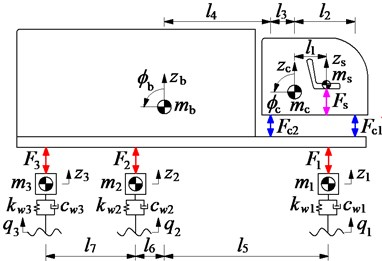a) The vehicle dynamic model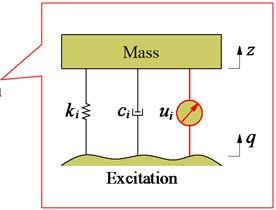b) Semi-active isolation systems

The dynamic reaction forces ${F}_{i}$, and ${F}_{wj}$ of the suspension systems of the seat, cab, vehicle, and wheels are respectively written by:

(1)
$\begin{array}{ll}{F}_{s}=& {k}_{s}\left({z}_{s}-{z}_{c}-{l}_{1}{\varphi }_{c}\right)+{c}_{s}\left({\stackrel{˙}{z}}_{s}-{\stackrel{˙}{z}}_{c}-{l}_{1}{\stackrel{˙}{\varphi }}_{c}\right)\end{array}+{u}_{s},$
(2)
${F}_{cn}={k}_{cn}\left[{z}_{c}-{z}_{b}+\left(-1{\right)}^{n}{l}_{n+1}{\varphi }_{c}+{l}_{p}{\varphi }_{b}\right]+{c}_{cn}\left[{\stackrel{˙}{z}}_{c}-{\stackrel{˙}{z}}_{b}+\left(-1{\right)}^{n}{l}_{n+1}{\stackrel{˙}{\varphi }}_{c}+{l}_{p}{\stackrel{˙}{\varphi }}_{b}\right]+{u}_{cn},$
(3)
${F}_{j}={k}_{j}\left[{z}_{b}-{z}_{j}+\left(-1{\right)}^{m}{l}_{q}{\varphi }_{b}\right]+{c}_{j}\left[{\stackrel{˙}{z}}_{b}-{\stackrel{˙}{z}}_{j}+\left(-1{\right)}^{m}{l}_{q}{\stackrel{˙}{\varphi }}_{b}\right]+{u}_{j},$
(4)
${F}_{wj}={k}_{wj}\left[{z}_{j}-{q}_{wj}\right]+{c}_{wj}\left[{\stackrel{˙}{z}}_{j}-{\stackrel{˙}{q}}_{wj}\right],$

where $n=$ 1-2, ${l}_{p}=$${l}_{2}+{l}_{3}+{l}_{4}$; when $j=$ 1 then $m=$ 1, ${l}_{p}={l}_{5}$; when $j=$ 2 then $m=$ 1, ${l}_{p}={l}_{4}$; when $j=$ 3 then $m=$ 2, ${l}_{p}={l}_{6}+{l}_{7}$.

Based on the vehicle dynamic model in Fig. 1, and by applying Newton’s second law of motion, the motion equations of the vehicle can be represented in the matrix form as follows:

(5)
$\left[\mathbf{M}\right]\left\{\stackrel{¨}{\mathbf{Z}}\right\}+\left[\mathbf{C}\right]\left\{\stackrel{˙}{\mathbf{Z}}\right\}+\left[\mathbf{K}\right]\left\{\mathbf{Z}\right\}=\left\{\mathbf{F}\left(t\right)\right\}$

where [M], [C] and [K] are ($a$×$a$) mass, damping and stiffness matrices, respectively, {$\mathbf{Z}$} is the ($a$×1) displacement vector, {$F\left(t\right)$} is the ($a$×1) exciting force vector, and $a$ is the number of DOF., ($a=$ 8).

#### 2.2. Applying the fuzzy logic controller for semi-active isolation systems

In order to evaluate the ride comfort performance with three types of semi-active isolation systems of the heavy truck, three types of the control suspensions including the seat controlled (${u}_{s}$), the cab controlled (${u}_{c1,c2}$), and the vehicle controlled (${u}_{1,2,3}$) are respectively given by:

(6)

Therefore, the different fuzzy logic controllers should be designed. However, design process of these controllers is same, thus a specific fuzzy control is designed. The design of a FLC is as follows: The deflection of the isolation systems “${E}_{i}$” and its derivation “” are the two input variables while the variable damping forces “${u}_{i}$” is the output value of the control isolation systems. The linguistic variables of input and output variables are defined by the positive big (PB), positive small (PS), zero (ZO), negative small (NS), and negative big (NB); and the membership functions for their variables are represented by a fuzzy set. The shape of membership functions is the Triangular function and their values are between 0 and 1, both input and output values are belonging to [–1, 1].

In this FLC, the if-then rules base are applied to describe according to expertise experiences and the designer’s knowledge, there are at most 25 possible rules, the fuzzy rules are given in Table 1, and written as follows ${R}_{i\alpha }$: If ${E}_{i\alpha }={A}_{i\alpha }$ and $E{C}_{i\alpha }={B}_{i\alpha }$ then ${u}_{i\alpha }={C}_{i\alpha }$, ($\alpha =$ 1-25).

The fuzzy inference system is selected by the minimum function and the centroid method of Mamdani . In this study, we used the FIS of Mamdani for control system model.

Table 1. Rule base of the FLC

 ${u}_{i}$ ${E}_{i}$ NB NS Z PS PB $E{C}_{i}$ NB PB PB PB PS ZE NS PB PS PS ZE NS Z PB PS ZE NS NB PS PS ZE NS NS NB PB ZE NS NB NB NB

Table 2. The weighted RMS acceleration values

 Type of control ${a}_{ws}$ / m.s-2 ${a}_{w\varphi c}$ / rad.s-2 Uncontrolled 0.7402 0.1536 Seat controlled 0.5076 0.1427 Cab controlled 0.5584 0.1070 Vehicle controlled 0.5993 0.1210

#### 2.3. Excitation vibration sources

In order to analyze the ride comfort performance of heavy truck with different control cases of semi-active isolation systems, two types of excitation road including the step and random road surfaces are considered as follows:

The excitation of step road: The set of typical step road disturbances be of the form:

(7)

The excitation of a random road: A random road surface represented by a randomly modulated periodic is carried out in this study. The general form of the displacement power spectral density (PSD) of the road surface is determined by the experimental formula :

(8)
${S}_{q}\left(n\right)={S}_{q}\left({n}_{0}\right){\left(\frac{n}{{n}_{0}}\right)}^{-\omega },$

where $n$ is space frequency; ${n}_{0}$ is reference space frequency, ${n}_{0}=$ 0.1 m-1; ${S}_{q}\left(n\right)$ is the PSD of the road surface under the reference space frequency ${n}_{0}$; $\omega$ is the frequency index which determines the frequency configuration of the PSD of the road surface ($\omega =$ 2 rad.s-1). The random road is assumed to be a zero-mean stationary Gaussian random process, and it can be generated by the Fourier transformation as follows:

(9)
$q\left(t\right)={\sum }_{i=1}^{N}\sqrt{2{S}_{q}\left({n}_{i}\right)\mathrm{\Delta }n}\mathrm{c}\mathrm{o}\mathrm{s}\left(i\mathrm{\Delta }\omega t+{\varphi }_{i}\right),$

where $N$ is the number of intervals, $\mathrm{\Delta }n=2\pi /L$ and $L$ is the length of the road segment, ${\varphi }_{i}$ is a random phase uniformly distributed from 0 to 2$\pi$, and $\mathrm{\Delta }\omega =\mathrm{\Delta }n{v}_{0}$ is the fundamental temporal frequency, ${v}_{0}$ is the vehicle speed.

Time histories of the excitation vibrations at speed ${v}_{0}=$ 20 m.s-1 are shown in Fig. 2.

Fig. 2. Time history of the excitation road sources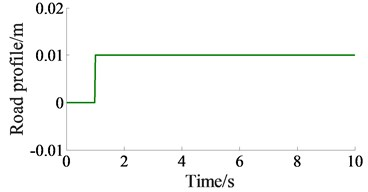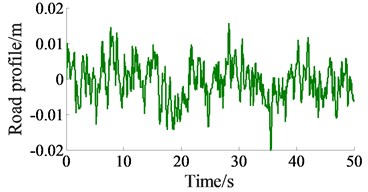b) Random road surface of ISO level C 

#### 3. Simulation results and analysis

The main objective of this study is to compare the performance of the various control cases of semi-active isolation systems under two different excitations of the road surface. In order to simulate and evaluate the results, Matlab R2015b/Simulink software, the conference parameters of a heavy truck , and a vehicle speed ${v}_{0}=$ 20 m.s-1 are chosen to evaluate the results.

#### 3.1. The performance on a step road surface

Three control cases of the semi-active isolation systems including the seat controlled, the cab controlled, and the vehicle controlled are respectively simulated under the condition of the vehicle traveling on a step road surface. The simulation results of the acceleration responses of the vertical driver’s seat and cab’s pitch angle are plotted in Fig. 3(a) and 3(b).

With the seat controlled, the simulation results are shown that the acceleration response of the vertical driver’s seat is greatly reduced in comparison with uncontrolled. However, the acceleration response of the cab’s pitch angle is insignificantly improved because the only ability of the seat controlled is to control the vibration in the vertical direction of the seat.

With the vehicle controlled, both the acceleration responses of the driver’s seat and cab’s pitch angle are significantly improved in comparison with uncontrolled. Besides, the acceleration responses of the driver’s seat and cab’s pitch angle with the cab controlled are strongly reduced comparable with both the vehicle controlled and uncontrolled. Therefore, in order to enhance the vertical ride comfort of the driver’s seat, the seat controlled should be used, whereas, in order to improve the vertical ride comfort of the driver’s seat and controlling the cab’s shake, the cab controlled or the vehicle controlled should be used.

Fig. 3. The acceleration responses under the excitation of a step road surface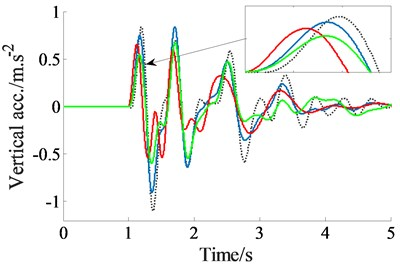a) The vertical driver’s seat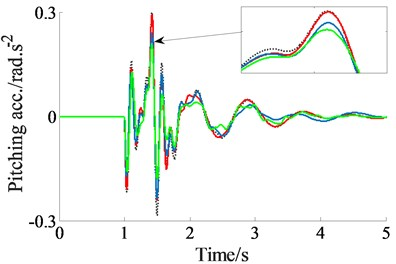b) The cab’s pitch angle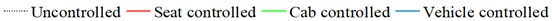#### 3.2. The performance on a random road surface

In the condition of the vehicle traveling on a road roughness surface, the simulation results of the acceleration responses of the vertical driver’s seat and cab’s pitch angle with three control cases are shown in Fig. 4(a) and 4(b). The comparison results are also similar in the condition of the vehicle traveling on a step road surface. The vertical driver’s seat is clearly improved by the seat controlled whereas both the vertical driver’s seat vibration and the cab’s shake are greatly reduced with the cab controlled.

Fig. 4. The acceleration responses under the excitation of a random road surface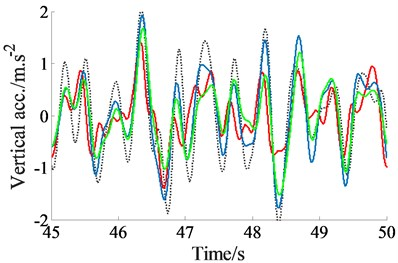a) The vertical driver’s seat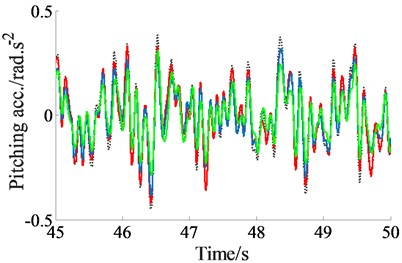b) The cab’s pitch angleAccording to the international standard ISO 2631-1, the effect of vibration on ride comfort is evaluated based on the weighted root-mean-square (RMS) acceleration responses (${a}_{wz}$) . The weighted RMS values of the vertical driver’s seat (${a}_{ws}$) and the cab’s pitch angle (${a}_{w\varphi c}$) are listed in Table 2. Comparing with uncontrolled, the ${a}_{ws}$ and ${a}_{w\varphi c}$ are decreased by 31.4 % and 7.1 % with the seat controlled, decreased by 24.5 % and 30.3 % with the cab controlled, and decreased by 19.0 % and 21.2 % with the vehicle controlled. Therefore, both ${a}_{ws}$ and ${a}_{w\varphi c}$ values of the cab controlled are greatly reduced. The simulation and analysis results show that all the heavy vehicles should be controlled by the cab’s suspension systems or the vehicle’s suspension system to improve the vehicle ride comfort. This is the reason that most heavy truck’s cab suspension systems are researched and controlled [1, 10]. Besides, the road friendliness of the heavy trucks is being concerned about using the vehicle’s air suspension systems or the vehicle’s semi-active suspension systems [3-6].

#### 4. Conclusions

The performance of the vehicle’s ride comfort with three control cases is compared under two excitation conditions of the road surfaces. The comparison results show that the vehicle’s ride comfort is significantly improved by three control cases of the semi-active isolation systems. Especially, in two simulation studies, the vertical driver’s seat vibration and the cab shake are greatly decreased by using the cab controlled. Therefore, the cab controlled surpasses the other types of control for improving the driver’s ride comfort and controlling the cab shake.

#### Acknowledgements

The work described in this paper was supported by Thai Nguyen University of Technology for an International Joint Scientific Project (Code: T2016-LK01).

1. Li Pengfei, Ma Li, He Tianming A simulation study on vibration isolation of cab mount s in a commercial vehicle. Automotive Engineering, Vol. 27, Issue 6, 2005, p. 741-743. [Search CrossRef]
2. Jing Chen, Cao Xiaolin, Wang Dengfeng Matching and optimization of heavy commercial vehicle. Journal of Jilin University, Vol. 29, Issue 5, 2009, p. 1125-1127. [Search CrossRef]
3. Tsampardoukas Georgios, Stammers Charles W. Hybrid balance control of a magnetorheological truck suspension. Journal of Sound and Vibration, Vol. 317, 2008, p. 514-536. [Publisher]
4. Ieluzzi Michele, Turco Patrizio, Montiglio Mauro Development of a heavy truck semi-active suspension control. Control Engineering Practice, Vol. 14, Issue 3, 2006, p. 305-312. [Publisher]
5. Xie Zhengchao, Wong Pak Kin, Zhao Jing, Xu Tao, Wong Ka In, Wong Hang Cheong A noise-insensitive semi-active air suspension for heavy-duty vehicles with an integrated fuzzy-wheelbase preview control. Mathematical Problems in Engineering, Vol. 2013, 2013, p. 121953. [Publisher]
6. Nguyen Van Liem, Zhang Jianrun, et al. Performance analysis of air suspension system of heavy truck with semi-active fuzzy control. Journal of Southeast University, Vol. 33, 2017, p. 159-165. [Search CrossRef]
7. Pekgökgöz R. K., et al. Active suspension of cars using fuzzy logic controller optimized by genetic algorithm. International Journal of Engineering and Applied Science, Vol. 2, 2010, p. 27-37. [Search CrossRef]
8. Yildirim Ş. Vibration control of suspension systems using a proposed neural network. Journal of Vibration and Control, Vol. 277, 2004, p. 1059-1069. [Publisher]
9. Herrán Félix L. C., De Rodríguez Ortizc J.-J., et al. Hinfcontrol of a suspension with a magnetorheological damper. International Journal of Control, Vol. 85, Issue 8, 2012, p. 1026-1038. [Publisher]
10. Nguyen Van Liem, Zhang Jianrun, et al. Study of fuzzy control for cab’s isolation system of heavy truck. Vibroengineering Procedia, Vol. 10, 2016, p. 309-314. [Search CrossRef]
11. Sy Dzung Nguyen, Quoc Hung Nguyen, Seung-Bok Choi A hybrid clustering based fuzzy structure for vibration control – part 2: an application to semi-active vehicle seat-suspension system. Mechanical Systems and Signal Processing, Vol. 450, Issues 56-57, 2015, p. 288-301. [Publisher]
12. Kasemi B., Muthalif A. G., Rashid M. M., Fathima S. Fuzzy-PID controller for semi-active vibration control using magnetorheological fluid damper. Procedia Engineering, Vol. 41, 2012, p. 1221-1227. [Publisher]
13. Qazi Abroon Jamal, et al. Performance analysis of a semi-active suspension system with particle swarm optimization and fuzzy logic control. The Scientific World Journal, Vol. 2014, 2014, p. 174102. [Publisher]
14. Mamdani E. H. Advances in the linguistic synthesis of fuzzy controllers. International Journal of Man-Machine Studies, Vol. 8, Issue 1, 1976, p. 669-678. [Publisher]
15. ISO 8068 Mechanical Vibration-Road Surface Profiles-Reporting of Measured Data. International Organization for Standardization, Geneve, Switzerland, 1995. [Search CrossRef]
16. Nguyen Van Liem, et al. Evaluating the effect of the working conditions on the ride comfort and road friendliness of the heavy trucks. Vibroengineering Procedia, Vol. 21, 2018, p. 83-88. [Publisher]

#### Cited By

 Lecture Notes in Networks and Systems Le Van Quynh, Bui Van Cuong, Hoang Anh Tan, Canh Chi Huan 2023 Journal of Wind Engineering and Industrial Aerodynamics Dragan Sekulic, Alexey Vdovin, Bengt Jacobson, Simone Sebben, Stian Moe Johannesen 2022 Journal of Wind Engineering and Industrial Aerodynamics Dragan Sekulic, Alexey Vdovin, Bengt Jacobson, Simone Sebben, Stian Moe Johannesen 2021 Lecture Notes in Networks and Systems Le Van Quynh, Bui Van Cuong, Le Xuan Long, Do Van Quan 2020